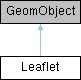Leaflet Class Reference

GeomObject representing a vertical leaflet that performs bending and stretching oscillations. More...

Inheritance diagram for Leaflet:## Public Member Functions

Leaflet (const double &length, const double &d_x, const double &d_y, const double &x_0, const double &period, Time *time_pt)
Constructor: Pass length (in Lagrangian coordinates), the amplitude of the horizontal and vertical deflection of the tip, the x-coordinate of the origin and the period of the oscillation. Passes the number of Lagrangian and Eulerian coordinates to the constructor of the GeomObject base class. More...

virtual ~Leaflet ()
Destructor – emtpy. More...

void position (const unsigned &t, const Vector< double > &xi, Vector< double > &r) const
Position vector, r, to the point identified by
its 1D Lagrangian coordinate, xi (passed as a 1D Vector) at discrete time level t (t=0: present; t>0: previous). More...

void position (const Vector< double > &xi, Vector< double > &r) const
Steady version: Get current shape. More...

unsigned ngeom_data () const
Number of geometric Data in GeomObject: None. More...

double length ()
Length of the leaflet. More...

double & d_x ()
Amplitude of horizontal tip displacement. More...

double d_y ()
Amplitude of vertical tip displacement. More...

double x_0 ()
x-coordinate of leaflet origin More...

## Private Attributes

double Length
Length in terms of Lagrangian coordinates. More...

double D_x
Horizontal displacement of tip. More...

double D_y
Vertical displacement of tip. More...

double X_0
Origin. More...

double T
Period of the oscillations. More...

Time * Time_pt
Pointer to the global time object. More...

## Detailed Description

GeomObject representing a vertical leaflet that performs bending and stretching oscillations.

Definition at line 43 of file channel_with_leaflet.cc.

## ◆ Leaflet()

 Leaflet::Leaflet ( const double & length, const double & d_x, const double & d_y, const double & x_0, const double & period, Time * time_pt )
inline

Constructor: Pass length (in Lagrangian coordinates), the amplitude of the horizontal and vertical deflection of the tip, the x-coordinate of the origin and the period of the oscillation. Passes the number of Lagrangian and Eulerian coordinates to the constructor of the GeomObject base class.

Definition at line 53 of file channel_with_leaflet.cc.

## ◆ ~Leaflet()

 virtual Leaflet::~Leaflet ( )
inlinevirtual

Destructor – emtpy.

Definition at line 60 of file channel_with_leaflet.cc.

## ◆ d_x()

 double & Leaflet::d_x ( )
inline

Amplitude of horizontal tip displacement.

Definition at line 88 of file channel_with_leaflet.cc.

References D_x.

## ◆ d_y()

 double Leaflet::d_y ( )
inline

Amplitude of vertical tip displacement.

Definition at line 91 of file channel_with_leaflet.cc.

References D_y.

## ◆ length()

 double Leaflet::length ( )
inline

Length of the leaflet.

Definition at line 85 of file channel_with_leaflet.cc.

References Length.

## ◆ ngeom_data()

 unsigned Leaflet::ngeom_data ( ) const
inline

Number of geometric Data in GeomObject: None.

Definition at line 82 of file channel_with_leaflet.cc.

## ◆ position() [1/2]

 void Leaflet::position ( const unsigned & t, const Vector< double > & xi, Vector< double > & r ) const
inline

Position vector, r, to the point identified by
its 1D Lagrangian coordinate, xi (passed as a 1D Vector) at discrete time level t (t=0: present; t>0: previous).

Definition at line 65 of file channel_with_leaflet.cc.

References D_x, D_y, Length, T, Time_pt, and X_0.

Referenced by position().

## ◆ position() [2/2]

 void Leaflet::position ( const Vector< double > & xi, Vector< double > & r ) const
inline

Definition at line 76 of file channel_with_leaflet.cc.

References position().

## ◆ x_0()

 double Leaflet::x_0 ( )
inline

x-coordinate of leaflet origin

Definition at line 94 of file channel_with_leaflet.cc.

References X_0.

## ◆ D_x

 double Leaflet::D_x
private

Horizontal displacement of tip.

Definition at line 102 of file channel_with_leaflet.cc.

Referenced by d_x(), and position().

## ◆ D_y

 double Leaflet::D_y
private

Vertical displacement of tip.

Definition at line 105 of file channel_with_leaflet.cc.

Referenced by d_y(), and position().

## ◆ Length

 double Leaflet::Length
private

Length in terms of Lagrangian coordinates.

Definition at line 99 of file channel_with_leaflet.cc.

Referenced by length(), and position().

## ◆ T

 double Leaflet::T
private

Period of the oscillations.

Definition at line 111 of file channel_with_leaflet.cc.

Referenced by position().

## ◆ Time_pt

 Time* Leaflet::Time_pt
private

Pointer to the global time object.

Definition at line 114 of file channel_with_leaflet.cc.

Referenced by position().

## ◆ X_0

 double Leaflet::X_0
private

Origin.

Definition at line 108 of file channel_with_leaflet.cc.

Referenced by position(), and x_0().

The documentation for this class was generated from the following file: